# Trees EENG 212 Algorithms and Data Structures Trees

• Slides: 39Trees EENG 212 Algorithms and Data Structures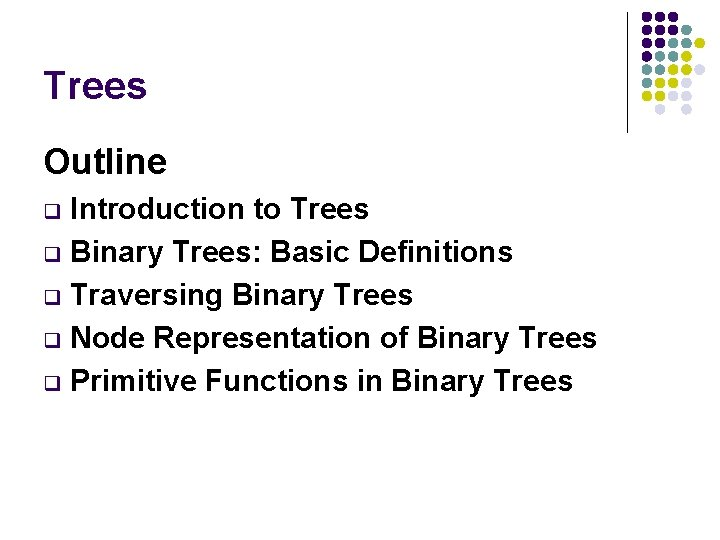Trees Outline Introduction to Trees q Binary Trees: Basic Definitions q Traversing Binary Trees q Node Representation of Binary Trees q Primitive Functions in Binary Trees q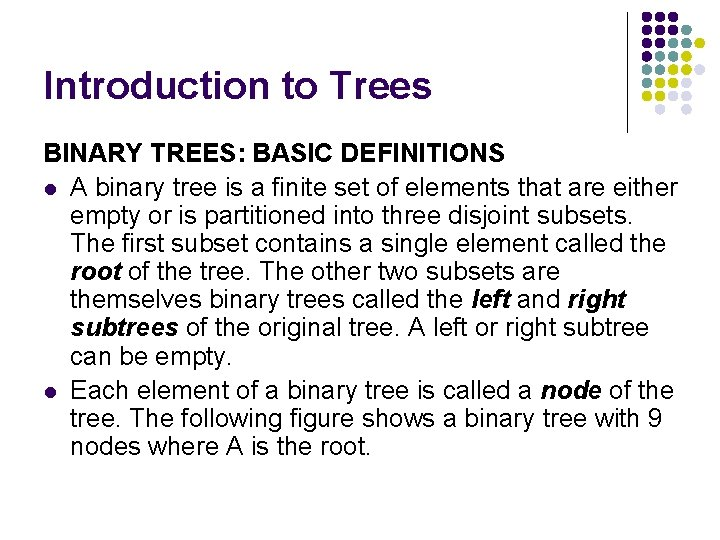Introduction to Trees BINARY TREES: BASIC DEFINITIONS l A binary tree is a finite set of elements that are either empty or is partitioned into three disjoint subsets. The first subset contains a single element called the root of the tree. The other two subsets are themselves binary trees called the left and right subtrees of the original tree. A left or right subtree can be empty. l Each element of a binary tree is called a node of the tree. The following figure shows a binary tree with 9 nodes where A is the root.BINARY TREES: BASIC DEFINITIONS root left subtree right subtree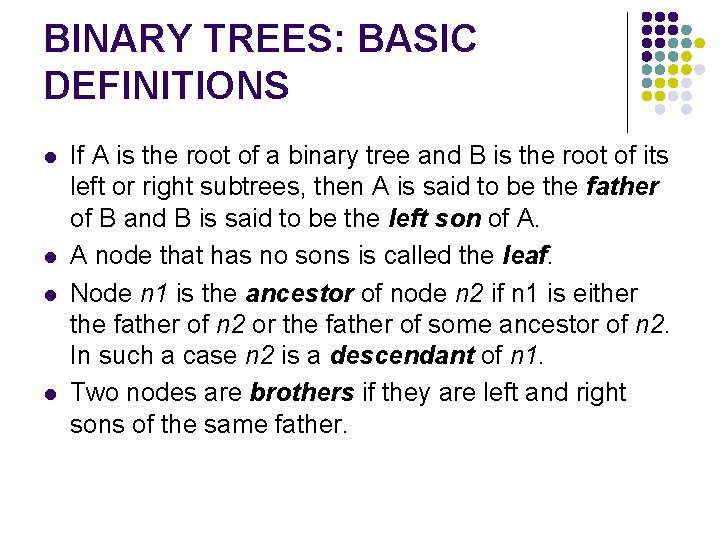BINARY TREES: BASIC DEFINITIONS l l If A is the root of a binary tree and B is the root of its left or right subtrees, then A is said to be the father of B and B is said to be the left son of A. A node that has no sons is called the leaf. Node n 1 is the ancestor of node n 2 if n 1 is either the father of n 2 or the father of some ancestor of n 2. In such a case n 2 is a descendant of n 1. Two nodes are brothers if they are left and right sons of the same father.BINARY TREES: BASIC DEFINITIONS left son right son leavesBINARY TREES: BASIC DEFINITIONS l If every nonleaf node in a binary tree has nonempty left and right subtrees, the tree is called a strictly binary tree.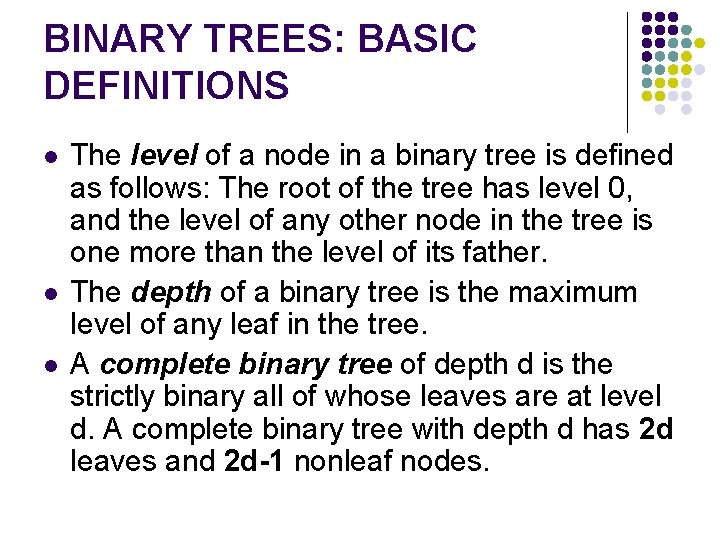BINARY TREES: BASIC DEFINITIONS l l l The level of a node in a binary tree is defined as follows: The root of the tree has level 0, and the level of any other node in the tree is one more than the level of its father. The depth of a binary tree is the maximum level of any leaf in the tree. A complete binary tree of depth d is the strictly binary all of whose leaves are at level d. A complete binary tree with depth d has 2 d leaves and 2 d-1 nonleaf nodes.BINARY TREES: BASIC DEFINITIONSTRAVERSING BINARY TREES l l One of the common operations of a binary tree is to traverse the tree. Traversing a tree is to pass through all of its nodes once. You may want to print the contents of each node or to process the contents of the nodes. In either case each node of the tree is visited. There are three main traversal methods where traversing a binary tree involves visiting the root and traversing its left and right subtrees. The only difference among these three methods is the order in which these three operations are performed.TRAVERSING BINARY TREES l Traversing a binary tree in preorder (depth-first order) 1. Visit the root. 2. Traverse the left subtree in preorder. 3. Traverse the right subtree in preorder.Traversing a binary tree in preorder Preorder: ABDGCEHIFTRAVERSING BINARY TREES Traversing a binary tree in inorder (or symmetric order) 1. Traverse the left subtree in inorder. 2. Visit the root. 3. Traverse the right subtree in inorder.Traversing a binary tree in inorder Inorder: DGBAHEICF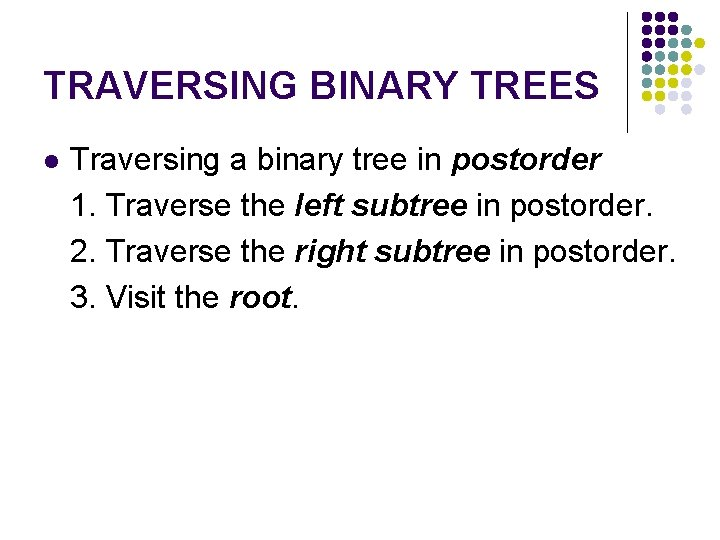TRAVERSING BINARY TREES l Traversing a binary tree in postorder 1. Traverse the left subtree in postorder. 2. Traverse the right subtree in postorder. 3. Visit the root.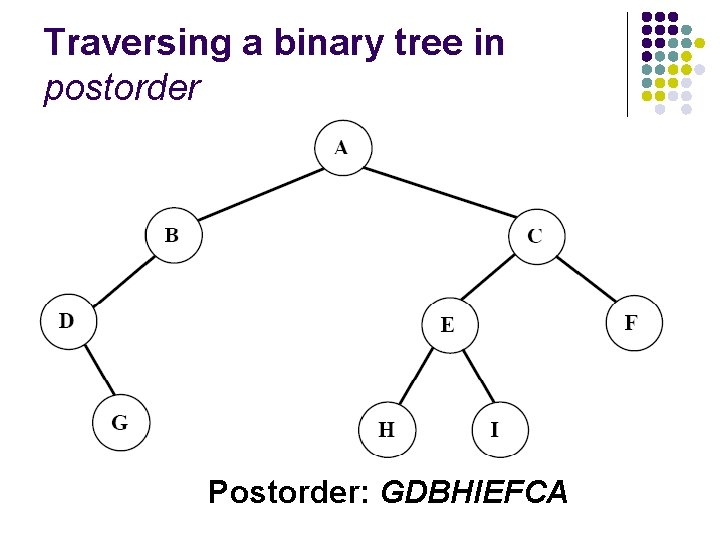Traversing a binary tree in postorder Postorder: GDBHIEFCA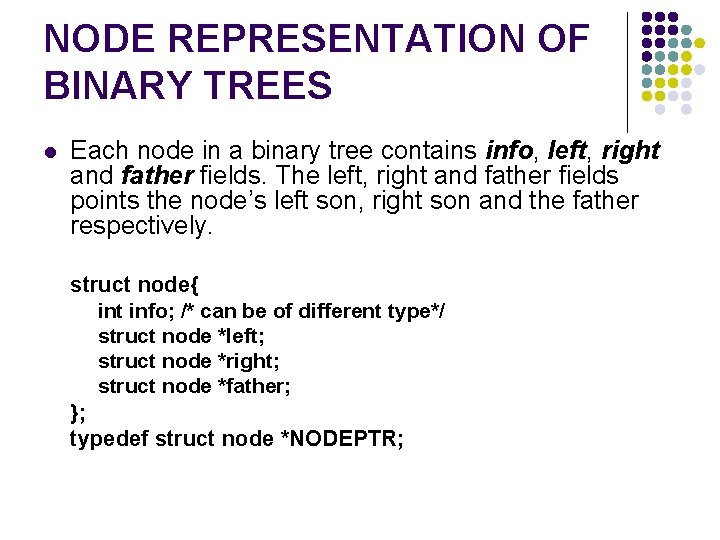NODE REPRESENTATION OF BINARY TREES l Each node in a binary tree contains info, left, right and father fields. The left, right and father fields points the node’s left son, right son and the father respectively. struct node{ int info; /* can be of different type*/ struct node *left; struct node *right; struct node *father; }; typedef struct node *NODEPTR;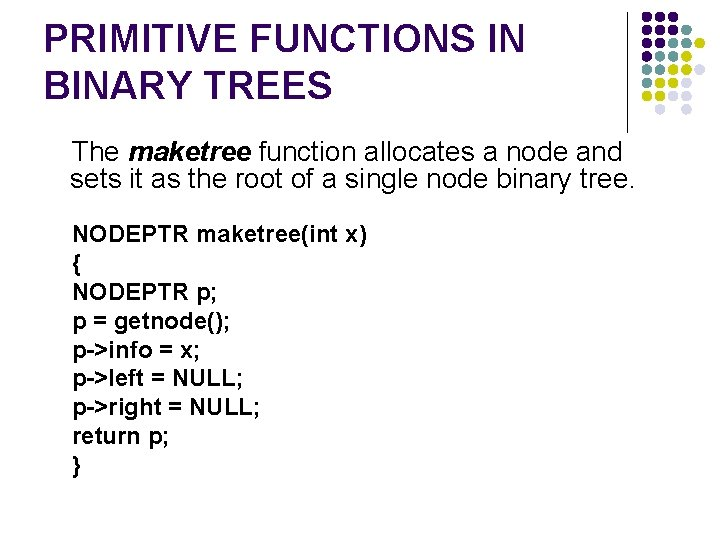PRIMITIVE FUNCTIONS IN BINARY TREES The maketree function allocates a node and sets it as the root of a single node binary tree. NODEPTR maketree(int x) { NODEPTR p; p = getnode(); p->info = x; p->left = NULL; p->right = NULL; return p; }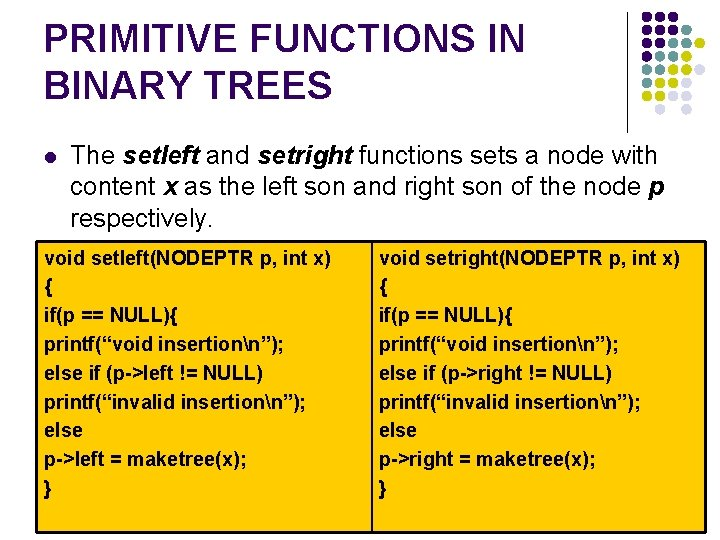PRIMITIVE FUNCTIONS IN BINARY TREES l The setleft and setright functions sets a node with content x as the left son and right son of the node p respectively. void setleft(NODEPTR p, int x) { if(p == NULL){ printf(“void insertionn”); else if (p->left != NULL) printf(“invalid insertionn”); else p->left = maketree(x); } void setright(NODEPTR p, int x) { if(p == NULL){ printf(“void insertionn”); else if (p->right != NULL) printf(“invalid insertionn”); else p->right = maketree(x); }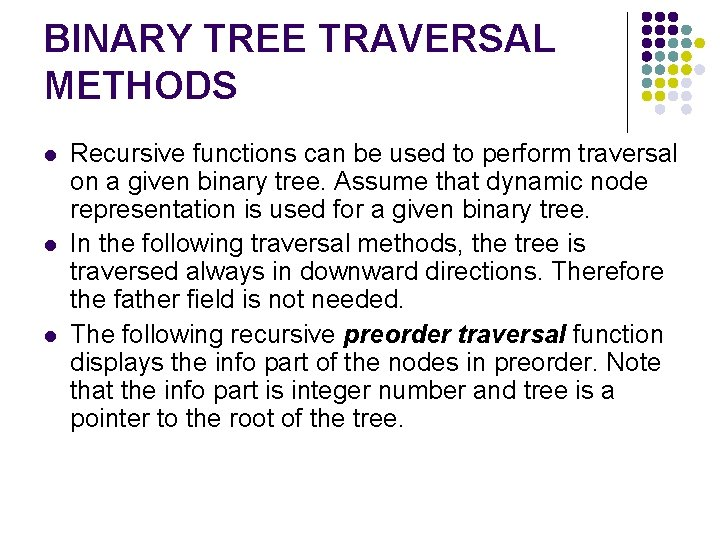BINARY TREE TRAVERSAL METHODS l l l Recursive functions can be used to perform traversal on a given binary tree. Assume that dynamic node representation is used for a given binary tree. In the following traversal methods, the tree is traversed always in downward directions. Therefore the father field is not needed. The following recursive preorder traversal function displays the info part of the nodes in preorder. Note that the info part is integer number and tree is a pointer to the root of the tree.BINARY TREE TRAVERSAL METHODS void pretrav(NODEPTR tree) { if(tree != NULL){ printf(“%dn”, tree->info); pretrav(tree->left); pretrav(tree->right); } }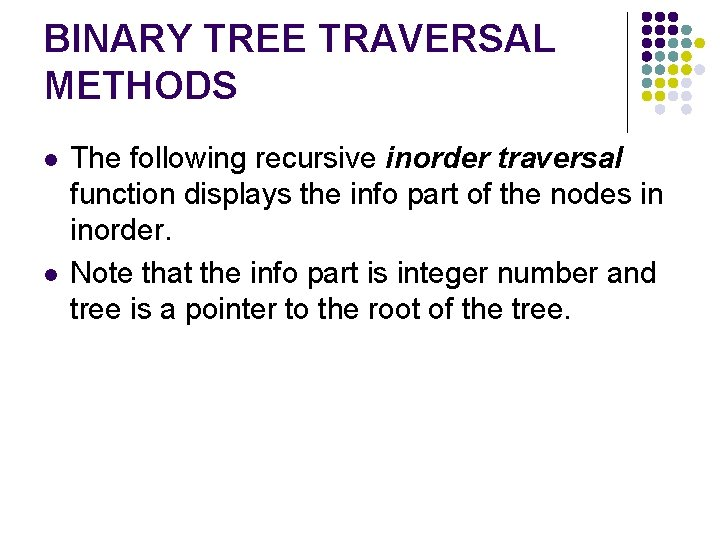BINARY TREE TRAVERSAL METHODS l l The following recursive inorder traversal function displays the info part of the nodes in inorder. Note that the info part is integer number and tree is a pointer to the root of the tree.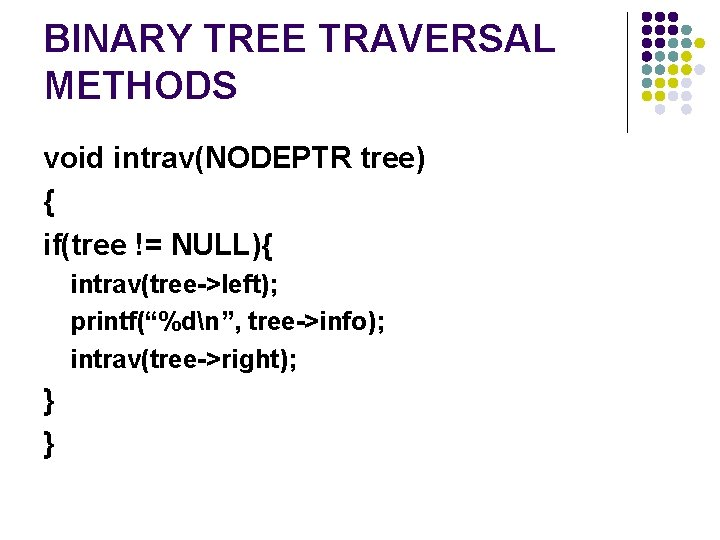BINARY TREE TRAVERSAL METHODS void intrav(NODEPTR tree) { if(tree != NULL){ intrav(tree->left); printf(“%dn”, tree->info); intrav(tree->right); } }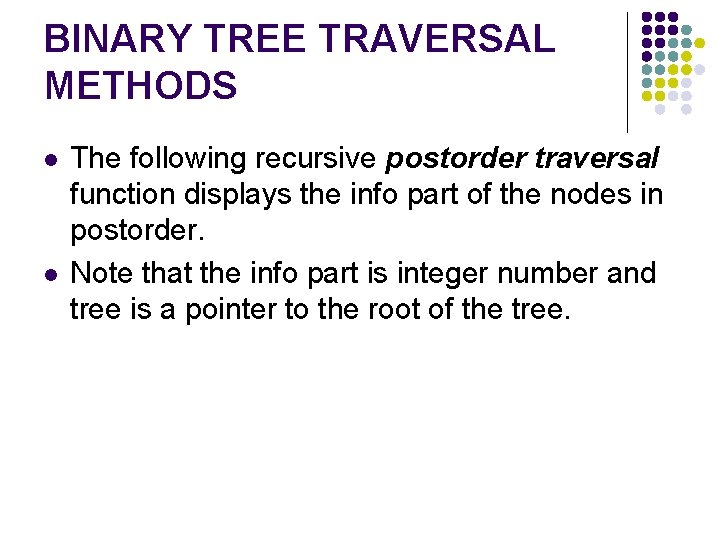BINARY TREE TRAVERSAL METHODS l l The following recursive postorder traversal function displays the info part of the nodes in postorder. Note that the info part is integer number and tree is a pointer to the root of the tree.BINARY TREE TRAVERSAL METHODS void posttrav(NODEPTR tree) { if(tree != NULL){ posttrav(tree->left); posttrav(tree->right); printf(“%dn”, tree->info); } }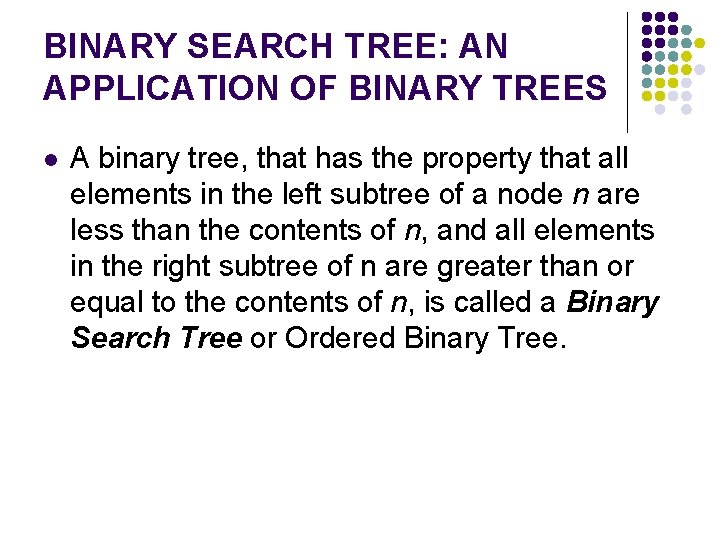BINARY SEARCH TREE: AN APPLICATION OF BINARY TREES l A binary tree, that has the property that all elements in the left subtree of a node n are less than the contents of n, and all elements in the right subtree of n are greater than or equal to the contents of n, is called a Binary Search Tree or Ordered Binary Tree.BINARY SEARCH TREE: AN APPLICATION OF BINARY TREES Given the following sequence of numbers, 14, 15, 4, 9, 7, 18, 3, 5, 16, 4, 20, 17, 9, 14, 5 l The following binary search tree can be constructed. lBINARY SEARCH TREE: AN APPLICATION OF BINARY TREES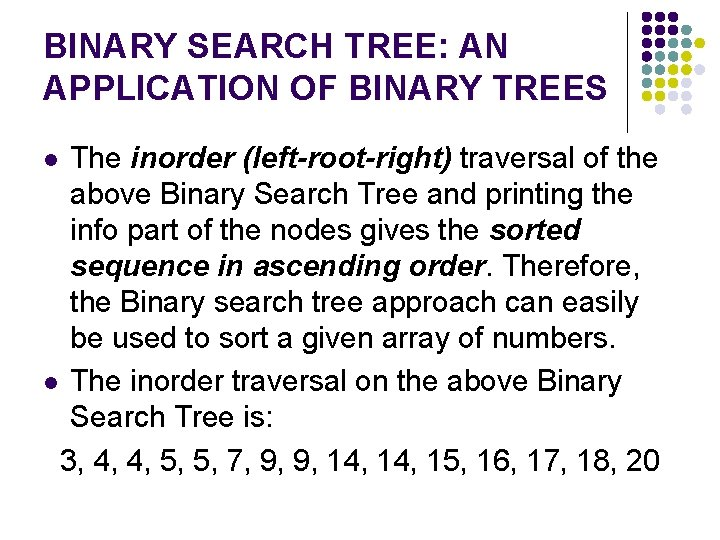BINARY SEARCH TREE: AN APPLICATION OF BINARY TREES The inorder (left-root-right) traversal of the above Binary Search Tree and printing the info part of the nodes gives the sorted sequence in ascending order. Therefore, the Binary search tree approach can easily be used to sort a given array of numbers. l The inorder traversal on the above Binary Search Tree is: 3, 4, 4, 5, 5, 7, 9, 9, 14, 15, 16, 17, 18, 20 lSEARCHING THROUGH THE BINARY SEARCH TREE l l Searching operation of the binary search tree is always in downward direction. Therefore the following node structure can be used to represent the node of a given binary search tree. Note that the father link is not required.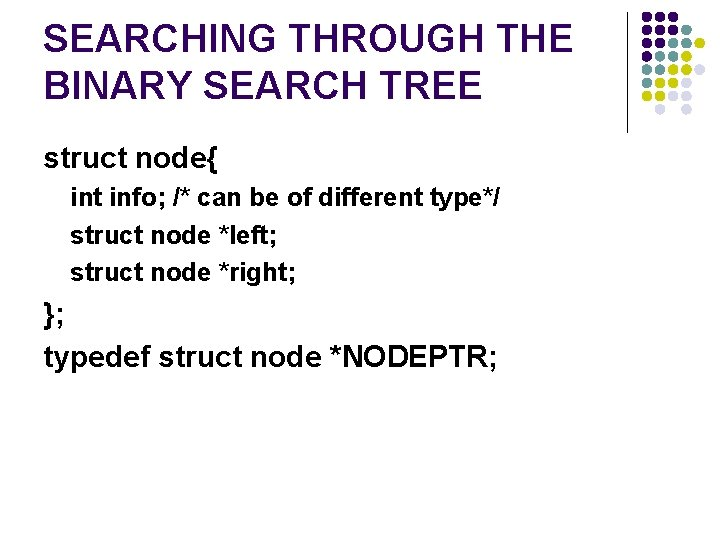SEARCHING THROUGH THE BINARY SEARCH TREE struct node{ int info; /* can be of different type*/ struct node *left; struct node *right; }; typedef struct node *NODEPTR;SEARCHING THROUGH THE BINARY SEARCH TREE l The following recursive function can be used to search for a given key element in a given array of integers. The array elements are stored in a binary search tree. Note that the function returns TRUE (1) if the searched key is a member of the array and FALSE (0) if the searched key is not a member of the array.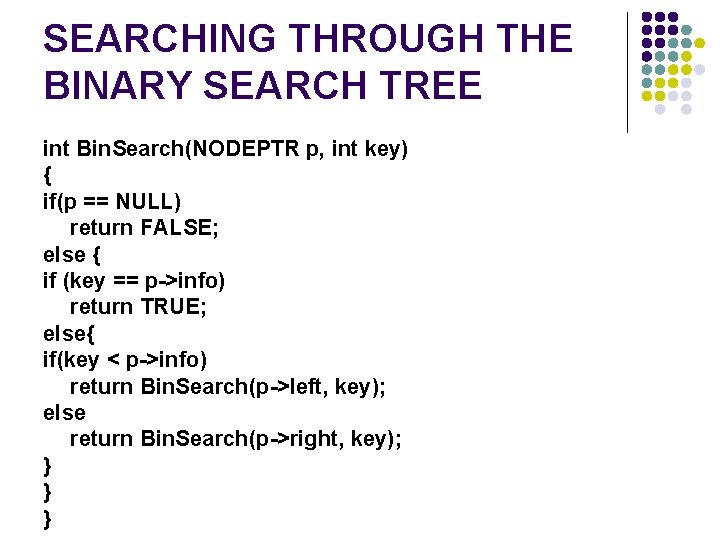SEARCHING THROUGH THE BINARY SEARCH TREE int Bin. Search(NODEPTR p, int key) { if(p == NULL) return FALSE; else { if (key == p->info) return TRUE; else{ if(key < p->info) return Bin. Search(p->left, key); else return Bin. Search(p->right, key); } } }INSERTING NODES INTO A BINARY SEARCH TREE l The following recursive function can be used to insert a new node into a given binary search tree.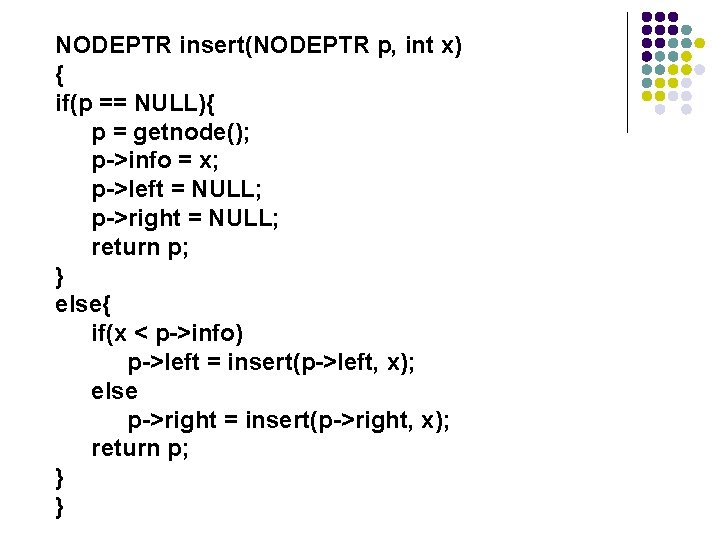NODEPTR insert(NODEPTR p, int x) { if(p == NULL){ p = getnode(); p->info = x; p->left = NULL; p->right = NULL; return p; } else{ if(x < p->info) p->left = insert(p->left, x); else p->right = insert(p->right, x); return p; } }Application of Binary Search Tree l Suppose that we wanted to find all duplicates in a list of numbers. One way of doing this to compare each number with all those that precede it. However this involves a large number of comparison. The number of comparison can be reduced by using a binary tree. The first number in the list is placed in a node that is the root of the binary tree with empty left and right sub-trees. The other numbers in the list is than compared to the number in the root. If it is matches, we have duplicate. If it is smaller, we examine the left sub-tree; if it is larger we examine the right subtree. If the sub-tree is empty, the number is not a duplicate and is placed into a new node at that position in the tree. If the sub-tree is nonempty, we compare the number to the contents of the root of the sub-tree and the entire process is repeated with the subtree. A program for doing this follows.#include <stdio. h> #include <stdlib. h> struct node { struct node *left ; int info ; struct node *right; }; typedef struct node *NODEPTR; NODEPTR maketree(int); NODEPTR getnode(void); void intrav(NODEPTR); void main() { int number; NODEPTR root , p , q; printf("%sn", "Enter First number"); scanf("%d", &number); root=maketree(number); /* insert first root item */ printf("%sn", "Enter the other numbers");while(scanf("%d", &number) !=EOF) { p=q=root; /* find insertion point */ while((number !=p->info) && q!=NULL) {p=q; if (number <p->info) q = p->left; else q = p->right; } q=maketree(number); /* insertion */ if (number==p->info) printf("%d is a duplicate n", number); else if (number<p->info) p->left=q; else p->right=q; } printf("Tree Created n "); /* inorder Traversing */ intrav(root); }void intrav(NODEPTR tree) { if(tree != NULL){ intrav(tree->left); printf(“%dn”, tree->info); intrav(tree->right); } } NODEPTR maketree(int x) { NODEPTR p; p = getnode(); p->info = x; p->left = NULL; p->right = NULL; return p; } NODEPTR getnode(void) { NODEPTR p; p=(NODEPTR) malloc(sizeof(struct node)); return p; }